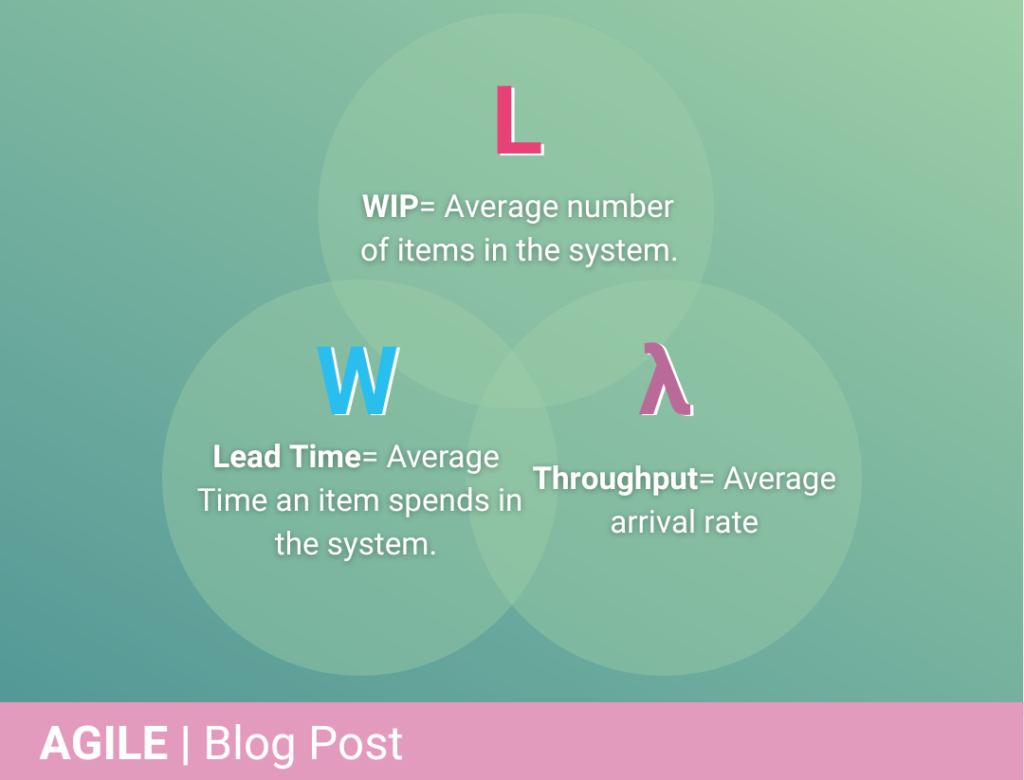# Little’s Law | Improve Process Performance

Learn How Little’s Law Can Improve Your Business Operations In A Big Way.

• Understand how Little’s Law works
• Integrate it into your Kanban framework
• Use assumptions to improve your estimations

## Little’s Law 101

We all want to make projects more efficient and streamlined. Is there a tried and tested way to get insights into how our project queues are structured, what faults they have and what we can do to improve them?

Little’s Law is a theorem for queuing systems which was developed in the 1960s. The law is based on a connection between three variables; the average arrival rate of a queue, the average number of items in the queue and the average amount of time an item spends in the queue.

Mathematically, the relationship between these three variables is the following:

L = λ * W

– L = Average number of items in the queue

– λ = Average arrival rate of items in the queue

– W = Average amount of time an item spends in the queue

What’s important to notice is that Little’s Law is a mathematical relationship of averages: Each of the three variables is affecting at least one or both other variables in the relationship. So, the law reveals what levers to pull when analyzing your process for any improvements.

For Little’s Law to hold, there is one assumption that needs to be valid, which is that fact that the system should be in a steady state. Meaning that the system stays constant over time.

## Adapting Little’s Law To Kanban

Little’s Law can be used for multiple applications, such as project management, software development, or manufacturing, but in this example we are focusing on how it can be applied to Kanban.

A different version of Little’s Law was developed in the 1980s which was focused on operations management. This version was more focused on the throughput and departure rate of a system rather than the arrival rate of a system. Because of the operations management roots, the Kanban community also adopted this throughput version. The operations management and Kanban community changed the Little’s Law into the following equation:

Work In Progress (WIP) = Throughput (TH) * Cycle time (CT)

– WIP = Average amount of items in WIP

– TH = Average throughput of the system

– CT = Average amount of time it takes for an item to flow through the system

In this equation there is a relationship between 3 variables in which each of them will affect one or both other variables.

Let’s jump into the technical stuff. If you have any questions, or if you are not familiar with Little’s Law, just get in touch and one of our technical team will reach out and help you understand how it can help your business.

## Sometimes, assumptions are everything

Looking at the two formulas mentioned above, it looks quite straightforward. This is unfortunately not the case. The difference between both the formulas is the focus of each one. The first formula focuses on the arrival rate, while the second while the second formula is concerned with the departure rate of the system. This makes the assumptions for the formulas different from each other. For the first formula, the only requirement is that your system is in a steady state. While the focus on throughput of the second formula requires a change in assumptions to be valid. The list of assumptions for this formula to hold is as follows:

1. The average input or arrival rate should be equal to the average output or departure rate.
2. All work that is started will eventually be completed and exit the system.
3. The amount of WIP should be roughly the same at the beginning and at the end of the time interval chosen for the calculation.
4. The average age of the WIP is neither increasing nor decreasing (WIP age is the time an item in progress has spent in the workflow until today).
5. Cycle time, WIP and throughput must all be measured using consistent units (e.g., days or hours).

These assumptions should be met for the time interval you are interested in for Little’s Law to be valid. Violating one or multiple of these assumptions decreases the correctness of the estimation of Little’s Law. Assumptions 1 and 2 are concerned with a conservation of Flow within the system. The assumptions 3 and 4 notion of the system’s stability and assumption 5 is necessary for the math of the law to come out correctly.

With Little’s Law, it is not important to focus on math and plugging in the numbers into the formula. The focus should be on the 5 assumptions mentioned above. The assumptions should be understood and well-known by your organization. Following these assumptions and tracking your system for these assumptions is what makes your system predictable. Breaking the assumptions will lead to increased unpredictability which can lead to longer cycle times and more process variability.

## The Bottom Line

Little’s Law is not about the mathematics of queueing theory, but it is about understanding the assumptions for the law to work. These assumptions can then be used as a guide, model or blueprint for the process policies. In case your current process is violating the assumptions of Little’s Law you lose the predictability of your system.

## Even More To Explore